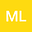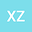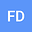General Lyapunov-Based Iterative Algorithm for Linear Quadratic Regulator Problem of Stochastic Systems with Markovian Jump
•••Xueyan Zhao
South China University of Technology
Author Profilefeiqi Deng
South China University of Technology
Author Profile## Abstract

This paper investigates the linear quadratic regulator(LQR) problem of linear stochastic systems with Markovian jump. Firstly, two iterative algorithms are proposed for solving the corresponding coupled algebraic Riccati equa- tions (CAREs) based on the general-type Lyapunov equation derived from linear stochastic systems. It is verified that the second algorithm adding an adjustable factor converges faster than the first one without it. Secondly, a monotonic convergence theorem is established for the proposed iterative algorithms under certain initial conditions. In the end, a numerical example is given to verify the efficiency of the proposed algorithms.